﻿ 6.10 EXERCISESoffice (412) 9679367
fax (412) 967-5958
toll-free 1 (800) 214-3480

D.5  Distribution of Stock Prices

In the geometric Brownian motion model of stock prices, the important assumptions made on the random variable, z, are:

1. The change in z is the product of a standard normal random variable (i.e., with mean zero and standard deviation 1) and the square root of the interval of time.  This implies that the variance of stock prices increases linearly with time.

2. For any two (non-overlapping and small) intervals of time, the change in z is independent.  This is consistent with the (weak form of the) efficient markets hypothesis.

In topic D.6, Derivation of Stock Price Distribution, we show that the log of the instantaneous stock return follows the diffusion process below: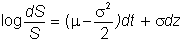Assumptions 1 and 2, together with the equation describing the log of the instantaneous stock return, give us a nice distribution for the stock price.  They imply that the random variable log(ST)-log(S), which is the change in the stock price between now and period T, is normally distributed with mean: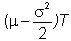and variance .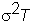Now, if X is a random variable and log(X) is normally distributed, then X itself has a lognormal distribution.  In fact, this is the definition of the lognormal distribution.  As a result, at the end of any interval of time, the stock price is lognormally distributed.  If log(X) is normally distributed with mean q and variance h2, then the mean and variance of X are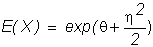.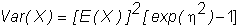For the case of the stock price, we have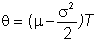and

.

Substituting for these values, we find that the mean of ST is:so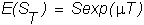and the variance of ST is: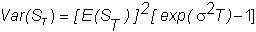In the next technical topic, Derivation of Stock Price Distribution, we formally show that the log of the instantaneous stock return follows the diffusion process shown.  This completes the characterization of the distributional properties for stock prices which are implied from the geometric Brownian motion model.